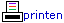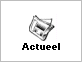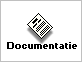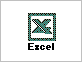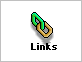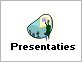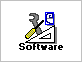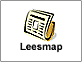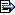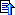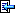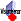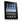## 2. Woordenlijst

Block
A 3x3 square of cells.

Buddies
Two cells that are in the same row, column or block.

Candidates
The possible digits that a cell can contain.

Cell
An individual square in the Sudoku where a digit can be placed.

Column
A vertical span of 9 cells. Columns are numbered 1 to 9, with the leftmost column being 1, and the rightmost column being 9.

Conjugates
Conjugates are a pair of cells in a unit which are the only two cells in that unit with a given candidate. One cell must contain the candidate, the other must not.

Grid
The complete set of cells in a Sudoku.

Intersection
The intersection of two cells is the set of cells that share a unit with both cells.

rXcY
The shorthand notation to refer to the cell in row X, column Y. For example, r2c4 is the cell in row 2, column 4.

Row
A horizontal span of 9 cells. Rows are numbered 1 to 9, with the top row being 1, and the bottom row being 9.

Unit
Each row, column, or block can be referred to as a unit.